# Cost function

The cost function associated with a given machine learning problem is a function that takes as input the predicted function value and actual observed output and associates to them a number measuring how far the predicted value is from the observed value.

## Definition

This section gives the definitions of cost functions for a single piece of data, for both regression problems and classification problems.

### Regression problems

For regression problems (prediction problems associated with continuous variables), both the predicted value and the actual value are continuous variables. The cost function is a function$C\colon \mathbb R \times \mathbb R \to \mathbb R$ of two variables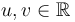$u,v \in \mathbb R$ (the predicted value and actual value) satisfying the following conditions:

•$C(u,u) = 0$ for all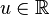$u \in \R$
• For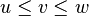$u \le v \le w$, we have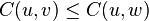$C(u,v) \le C(u,w)$ and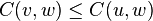$C(v,w) \le C(u,w)$

The cost function need not satisfy the triangle inequality; in fact, typical cost functions penalize bigger errors superlinearly.

Two typical examples of cost functions are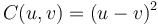$C(u,v) = (u-v)^2$ (the quadratic cost function, used for least squares linear regression) and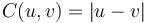$C(u,v) = |u-v|$ (used for least absolute deviations regression). With the former cost function, we see that for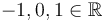$-1,0,1 \in \mathbb R$, we have$(-1-0)^2 + (0-1)^2 = 2 \not\geq 4 = (-1-1)^2$, so the triangle inequality is not satisfied.

### Binary classification problems

For binary classification problems (prediction problems associated with discrete variables), the predicted value is a probability and the actual value is simply a discrete value (0 or 1). The cost function is a function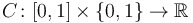$C\colon [0,1]\times \{0,1\} \to \mathbb R$ of two variables$p\in [0,1]$ and$v \in \{0,1\}$ (the predicted probability and actual value) satisfying the following conditions:

•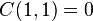$C(1,1) = 0$
•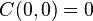$C(0,0) = 0$
• For$p \le q$, we have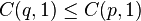$C(q,1) \le C(p,1)$
• For$p \le q$, we have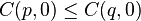$C(p,0) \le C(q,0)$

Although not a strict requirement, cost functions should be selected to be proper scoring rules, so that they penalize accurate probabilities less than inaccurate probabilities.

Typical cost functions used for binary classification problems include the logarithmic cost function and the quadratic cost function, both of which are proper scoring rules. Of these, the logarithmic scoring rules is the more widely used, because it is the only proper scoring rule when considering more than two classes.

## Combining the cost function values across multiple data points

To compute the cost function for several data points, we need to know the cost function for a single data point, as well as an approach for averaging the cost functions. The following are some typical choices:

• Arithmetic mean: This is the most common, and the default specification. This is equivalent to using the sum of the cost functions, but using the mean instead of the sum is preferable because that allows us to directly compare cost function values for data sets of different sizes.
• Mean using$r^{\text{th}}$ powers, for some$r > 1$: We take the mean of the$r^{\text{th}}$ powers of all the cost functions, then take the$r^{\text{th}}$ root.
• Maximum value

Note that there is some flexibility in terms of how we divide the load between the choice of cost function and the choice of averaging function: for instance, there is some equivalence between using the absolute value cost function and the root mean square averaging process versus using the squared error cost function and the arithmetic mean averaging process. The cost functions obtained in both cases are equivalent under a monotone transformation. If, however, we are considering adding regularization terms, then the distinction between the cost functions matters.